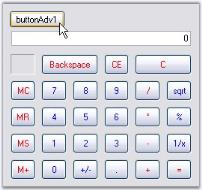# Popup Calculator in Windows Forms Calculator

26 Apr 20212 minutes to read

The PopupCalculator class can be used to display a popup Calculator control. This class can be created programmatically.

The PopupCalculator control lets you embed a Calculator control object to a button for example. Drop the button onto the form and add the following code snippet.

• c#
• ``````private Syncfusion.Windows.Forms.Tools.PopupCalculator popupCalculator1;

private void buttonAdv1_Click(object sender, EventArgs e)

{

// Create the Popup Calculator.

popupCalculator1 = new Syncfusion.Windows.Forms.Tools.PopupCalculator();

// The control that will act as the Popup's parent.

this.popupCalculator1.ParentControl = this.button1;

// Set the alignment.

this.popupCalculator1.PopupCalculatorAlignment = Syncfusion.Windows.Forms.Tools.CalculatorPopupAlignment.Right;

// Display the Calculator control.

this.popupCalculator1.DisplayCalculator(Point.Empty);

//Sets the size of the calculator

this.popupCalculator1.Size = this.calculatorControl1.Size;

}``````
• vbnet
• ``````Private popupCalculator1 As Syncfusion.Windows.Forms.Tools.PopupCalculator

Private Sub buttonAdv1_Click(ByVal sender As Object, ByVal e As EventArgs)

' Create the Popup Calculator.

popupCalculator1 = New Syncfusion.Windows.Forms.Tools.PopupCalculator()

' The control that will act as the Popup's parent.

Me.popupCalculator1.ParentControl = Me.button1

' Set the alignment.

Me.popupCalculator1.PopupCalculatorAlignment = Syncfusion.Windows.Forms.Tools.CalculatorPopupAlignment.Right

' Display the Calculator control.

Me.popupCalculator1.DisplayCalculator(Point.Empty)

'Sets the size of the calculator

Me.popupCalculator1.Size = Me.calculatorControl1.Size

End Sub``````